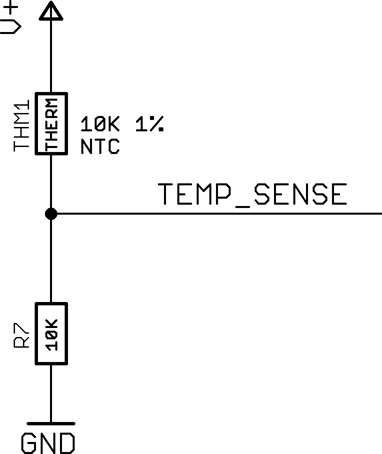## NTC Thermistor

An NTC (negative temperature coefficient)  thermistor is a resistor whose resistance decreases with increasing temperature. They are low cost and fairly accurate, and can be read by a simple analogue input.

Adafruit include a thermistor on their Circuit Playground Express  and Circuit Playground Bluefruit .

The thermistor is usually connected in a voltage divider arrangement with a fixed resistor:With this configuration the resistance of the thermistor is defined as follows, where reading is the 10-bit ADC reading, from 0 to 1023, and series-res is the series resistance, 10kΩ:

resistance = series-res × (
- 1)

The following program reads the thermistor and returns the temperature in °C. It uses the following parameters:

 Parameter Typical value Description pin 23 The ADC pin series-res 10000 The series resistor nom-res 10000 The resistance of the thermistor at the nominal temperature nom-temp 25 The nominal temperature in °C b-coeff 3380 The B coefficient of the thermistor, from the datasheet

The values above are suitable for the thermistor used in Adafruit's Circuit Playground.

```(defun temperature (pin series-res nom-res nom-temp b-coeff)
(analogreference :ar-vdd4)
(-
(/
(+
(/ (+ nom-temp 273.15))
(/
(log (/ (* series-res (1- (/ 1023 (analogread pin)))) nom-res))
b-coeff)))
273.15))```

For example, with the Circuit Playground Bluefruit you can read the temperature with:

```> (temperature 23 10000 10000 25 3380)
20.3813```

1. ^ Circuit Playground Express on Adafruit.
2. ^ Circuit Playground Bluefruit on Adafruit.

Previous: I2C Port Scanner